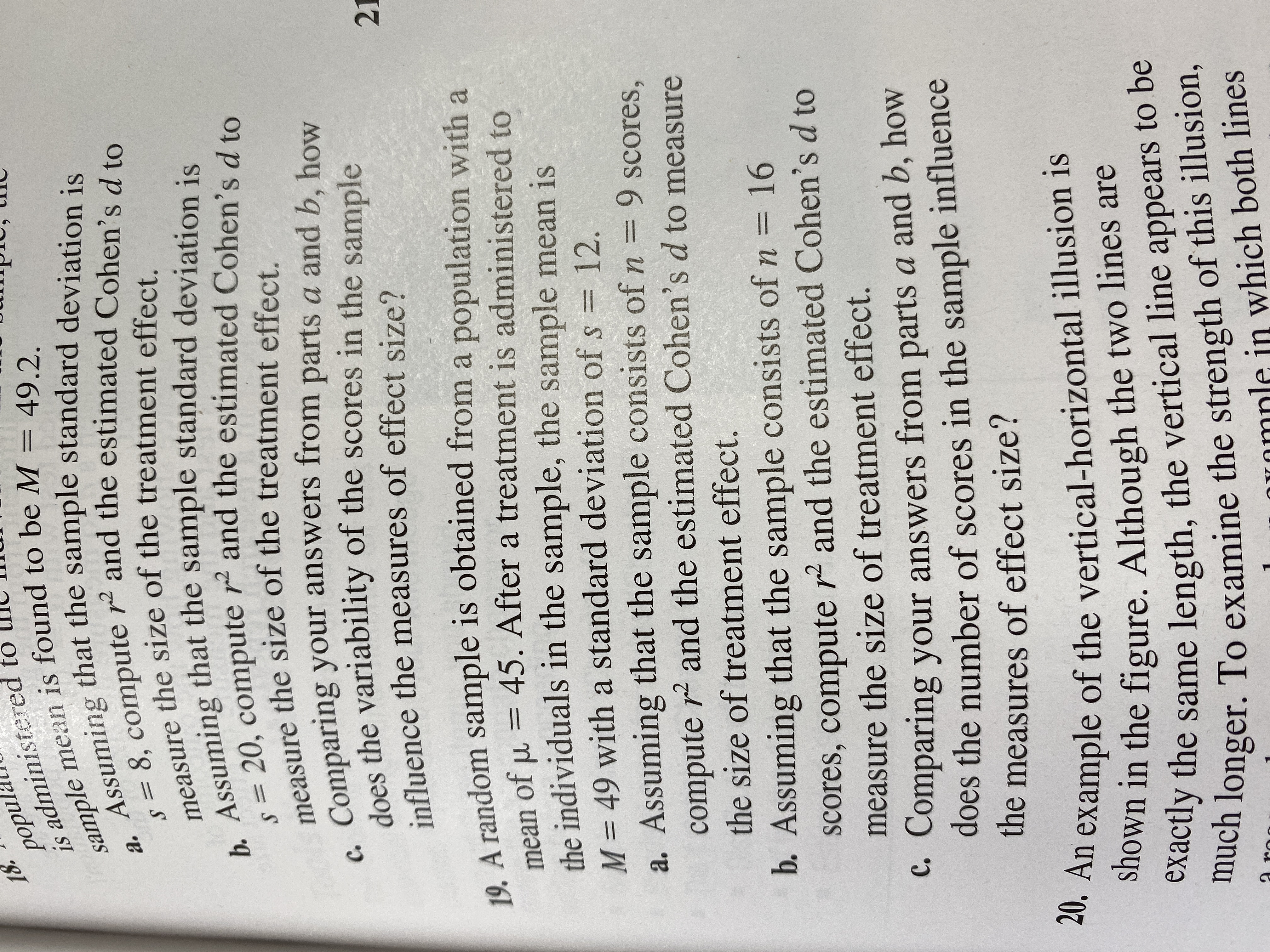# 18.рорul.is administered tosample mean is found to be M = 49.2.Assuming that the sample standard deviation is8, compute r and the estimated Cohen's d toa.measure the size of the treatment effect.b. Assuming that the sample standard deviation is20, compute r2 and the estimated Cohen's d tomeasure the size of the treatment effect.Comparing your answers from parts a and b, howdoes the variability of the scores in the sampleс.influence the measures of effect size?2119. A random sample is obtained from a population with a45. After a treatment is administered tomean of uthe individuals in the sample, the sample mean isM 49 with a standard deviation of s 12.a. Assuming that the sample consists of n = 9 scores,compute r2 and the estimated Cohen's d to measurethe size of treatment effect.b. Assuming that the sample consists of n =16scores, compute r2 and the estimated Cohen's d tomeasure the size of treatment effect.C. Comparing your answers from parts a and b, howdoes the number of scores in the sample influencethe measures of effect size?20. An example of the vertical-horizontal illusion isshown in the figure. Although the two lines areexactly the same length, the vertical line appears to bemuch longer. To examine the strength of this illusion,aroOnmnle in which both lines

Question
24 views

Number 19help_outlineImage Transcriptionclose18. рорul. is administered to sample mean is found to be M = 49.2. Assuming that the sample standard deviation is 8, compute r and the estimated Cohen's d to a. measure the size of the treatment effect. b. Assuming that the sample standard deviation is 20, compute r2 and the estimated Cohen's d to measure the size of the treatment effect. Comparing your answers from parts a and b, how does the variability of the scores in the sample с. influence the measures of effect size? 21 19. A random sample is obtained from a population with a 45. After a treatment is administered to mean of u the individuals in the sample, the sample mean is M 49 with a standard deviation of s 12. a. Assuming that the sample consists of n = 9 scores, compute r2 and the estimated Cohen's d to measure the size of treatment effect. b. Assuming that the sample consists of n =16 scores, compute r2 and the estimated Cohen's d to measure the size of treatment effect. C. Comparing your answers from parts a and b, how does the number of scores in the sample influence the measures of effect size? 20. An example of the vertical-horizontal illusion is shown in the figure. Although the two lines are exactly the same length, the vertical line appears to be much longer. To examine the strength of this illusion, aro Onmnle in which both lines fullscreen
check_circle

Step 1

It is given that s is 12, µ is 45 and M is 49.

a)

Here, n is 9.

The formula for finding the value of r2 is obtained as follows:

Step 2

The value of t is obtained as follows:

Step 3

Substitute the values of t an...

### Want to see the full answer?

See Solution

#### Want to see this answer and more?

Solutions are written by subject experts who are available 24/7. Questions are typically answered within 1 hour.*

See Solution
*Response times may vary by subject and question.
Tagged in